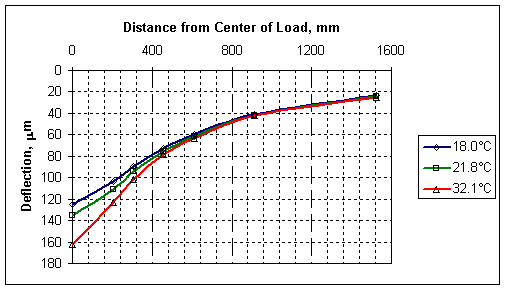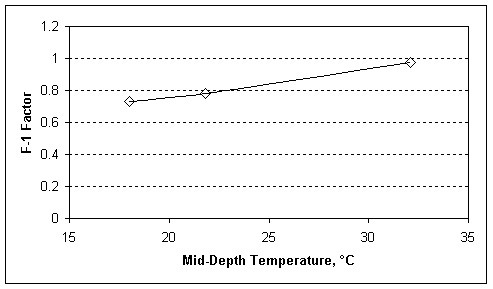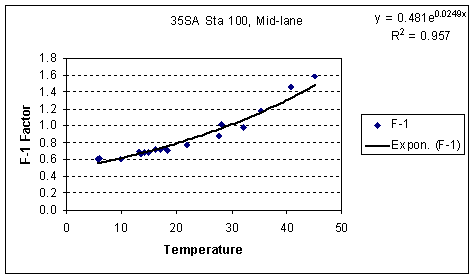U.S. Department of Transportation
1200 New Jersey Avenue, SE
Washington, DC 20590
202-366-4000

Federal Highway Administration Research and Technology
Coordinating, Developing, and Delivering Highway Transportation InnovationsThis report is an archived publication and may contain dated technical, contact, and link information
 Federal Highway Administration > Publications > Research Publications > LTPP Publications >   F1.Cfm > LTPP Guide to Asphalt Temperature Prediction and Correction
 Publication Number: FHWA-RD-98-085

# LTPP Guide to Asphalt Temperature Prediction and Correction

The F-1 factor is calculated by dividing the difference between defl0 and defl24 by defl12. The equation for calculating the F-1 factor is:

F1=(defl0 - defl24)/defl12

The F-1 basin shape factor relates to the ratio of bound layer stiffness to the unbound layer and subgrade stiffness. The bound layer stiffness characteristic here is dependent on both the thickness, and on the stiffness of the materials that make up the bound layer. The role that stiffness plays is clearly shown in Figure 19 by the relationship between F-1 factor and pavement temperature. Increasing the thickness of the asphalt would decrease the F-1 factor, whereas decreasing the thickness would increase the F-1 factor. Likewise, if this same pavement structure was placed on a less stiff subgrade, it would decrease the F-1 factor, or conversely, if the subgrade was stiffer, the F-1 would increase. F-1 values can range from about 0.3 for very stiff thick asphalt pavements on soft subgrade soils to the low 2's for thin soft asphalt pavements on stiff subgrade soils. A basin calculated for deflections on a one-layer system about 3.5. If there is a hard bottom, the F-1 factor will be higher, increasing as the surface gets closer to the hard bottom.

The F-1 basin factor increases with the mid-depth temperature of the asphalt, that is, as the temperature goes up, the magnitude of F-1 goes up. The relationship between the mid-depth temperature of the asphalt and F-1 best follows a semi-log relationship. Figure 19 shows three basins measured at the same spot, on the same day as the asphalt temperature rose. Figure 20 shows the F-1 factors that relate to the basins in Figure 19 and Figure 21 shows the F-1 factors at this same point over one year of testing during the LTPP Seasonal Monitoring Program.Figure 19. Sample Deflection Basins Measured at the Same PointFigure 20. F-1 Factors of Basins in Figure 17Figure 21. F-1 Factors Versus Temperature at a Single Test Location

The LTPP SMP data was used to develop a regression equation that relates the F-1 basin factor to pavement characteristics including the thickness of the asphalt, the temperature at the mid-depth of the asphalt, the 9 kip deflection at the 36-inch offset (which provides a replacement to the subgrade stiffness), and the latitude. (The latitude relates to the stiffness of the asphalt binder used in the mix. Hot southern climates use a stiffer binder than cooler or colder northern climates, which generally results in the asphalt mixes in colder climates being softer than asphalt mixes in warmer climates.)

The resulting regression equation for the F-1 basin factor is as follows:

log(F1) = 0.326 - 0.382*log(ac)*log(defl36) + 0.327*log(lat)*log(defl36) - 0.004447*T + 0.00555*T*log(ac)

Where:

ac = Total thickness of the HMA, mm

lat = Latitude of the pavement section

defl36 = Deflection (load-normalized to 40.5 kN (9 kip)) at 915 mm (36") from the center of the load plate, µm

T = Temperature at mid-depth of the HMA, °C

Source code for implementing this equation as a function in MS Excel VBA is available here.

Sample data for checking code is available here.

The F-1 factor decreases as the log of the thickness of the ac increases; increase as the temperature of the asphalt increases because the second term with T dominates the first term with T. The latitude is a substitute for the stiffness of the binder used in the asphalt mix and the F-1 increases as the latitude increases because softer binders are used in north and harder binders are used in the south. The coefficient on the term where the latitude appears is positive, indicating that as the latitude increases, the F-1 factor increases which is consistent with the relationship between the F-1 factor and stiffness. The defl36 variable is in two terms where the net result of an increase in defl36 is an decrease in the F-1 factor. The delta36 varies inversely with the subgrade stiffness, so as defl36 increases, the subgrade stiffness decreases and the ratio of the stiffness of the asphalt layer to the subgrade increases.

Use of the F-1 basin factor generally will require that the calculated F-1 values be adjusted for temperature. Temperature adjustment factors can be calculated by using the F-1 equation to calculate an F-1 for the pavement tested using the mid-depth asphalt temperature at the time of the test (Tm). The F-1 is then calculated again using the mid-depth asphalt temperature, or reference temperature (Tr) that it is to be adjusted to. The Basin Adjustment Factor for F-1, or BAFF-1, is the F-1 at the reference temperature divided by the F-1 at the measured temperature. The functions can be used to build tables of adjustment factors, or to calculate adjustment factors on a case-by-case basis.

Source code for implementing BAFF1 as a function in MS Excel VBA is available here.

Sample data for checking code is available here.